# How to Draw Organic Molecules

•• Contributed by Jim Clark
• Former Head of Chemistry and Head of Science at Truro School in Cornwall

This page explains the various ways that organic molecules can be represented on paper or on screen - including molecular formulae, and various forms of structural formulae.

## Molecular formulae

A molecular formula simply counts the numbers of each sort of atom present in the molecule, but tells you nothing about the way they are joined together. For example, the molecular formula of butane is $$C_4H_{10}$$, and the molecular formula of ethanol is $$C_2H_6O$$.

Molecular formulae are very rarely used in organic chemistry, because they do not give useful information about the bonding in the molecule. About the only place where you might come across them is in equations for the combustion of simple hydrocarbons, for example:

$C_5H_{12} + 8O_2 \rightarrow 5CO_2 + 6H_2O$

In cases like this, the bonding in the organic molecule isn't important.

## Structural formulae

A structural formula shows how the various atoms are bonded. There are various ways of drawing this and you will need to be familiar with all of them.

## Displayed formulae

A displayed formula shows all the bonds in the molecule as individual lines. You need to remember that each line represents a pair of shared electrons. For example, this is a model of methane together with its displayed formula: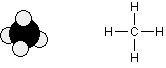Notice that the way the methane is drawn bears no resemblance to the actual shape of the molecule. Methane isn't flat with 90° bond angles. This mismatch between what you draw and what the molecule actually looks like can lead to problems if you aren't careful. For example, consider the simple molecule with the molecular formula CH2Cl2. You might think that there were two different ways of arranging these atoms if you drew a displayed formula.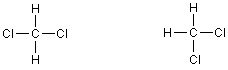The chlorines could be opposite each other or at right angles to each other. But these two structures are actually exactly the same. Look at how they appear as models.One structure is in reality a simple rotation of the other one. Consider a slightly more complicated molecule, C2H5Cl. The displayed formula could be written as either of these: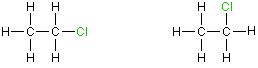But, again these are exactly the same. Look at the models.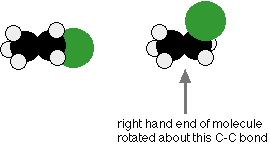## The commonest way to draw structural formulae

For anything other than the most simple molecules, drawing a fully displayed formula is a bit of a bother - especially all the carbon-hydrogen bonds. You can simplify the formula by writing, for example, CH3 or CH2 instead of showing all these bonds. For example, ethanoic acid would be shown in a fully displayed form and a simplified form as: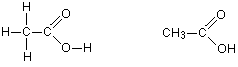You could even condense it further to CH3COOH, and would probably do this if you had to write a simple chemical equation involving ethanoic acid. You do, however, lose something by condensing the acid group in this way, because you can't immediately see how the bonding works. You still have to be careful in drawing structures in this way. Remember from above that these two structures both represent the same molecule:The next three structures all represent butane.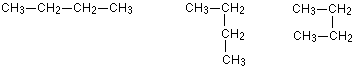All of these are just versions of four carbon atoms joined up in a line. The only difference is that there has been some rotation about some of the carbon-carbon bonds. You can see this in a couple of models.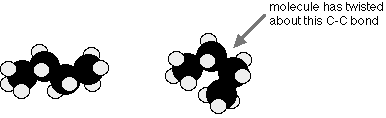Not one of the structural formulae accurately represents the shape of butane. The convention is that we draw it with all the carbon atoms in a straight line - as in the first of the structures above. This is even more important when you start to have branched chains of carbon atoms. The following structures again all represent the same molecule - 2-methylbutane.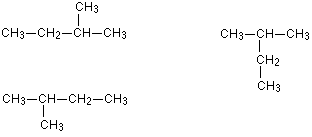The two structures on the left are fairly obviously the same - all we've done is flip the molecule over. The other one isn't so obvious until you look at the structure in detail. There are four carbons joined up in a row, with a CH3 group attached to the next-to-end one. That's exactly the same as the other two structures. If you had a model, the only difference between these three diagrams is that you have rotated some of the bonds and turned the model around a bit.

To overcome this possible confusion, the convention is that you always look for the longest possible chain of carbon atoms, and then draw it horizontally. Anything else is simply hung off that chain. It does not matter in the least whether you draw any side groups pointing up or down. All of the following represent exactly the same molecule.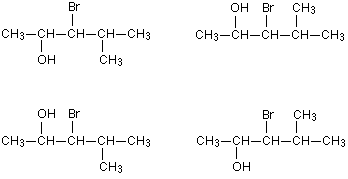If you made a model of one of them, you could turn it into any other one simply by rotating one or more of the carbon-carbon bonds.

## How to draw structural formulae in 3-dimensions

There are occasions when it is important to be able to show the precise 3-D arrangement in parts of some molecules. To do this, the bonds are shown using conventional symbols: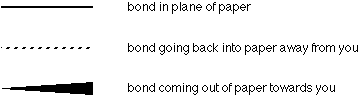For example, you might want to show the 3-D arrangement of the groups around the carbon which has the -OH group in butan-2-ol.

Example 1: butan-2-ol

Butan-2-ol has the structural formula: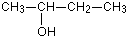Using conventional bond notation, you could draw it as, for example:The only difference between these is a slight rotation of the bond between the centre two carbon atoms. This is shown in the two models below. Look carefully at them - particularly at what has happened to the lone hydrogen atom. In the left-hand model, it is tucked behind the carbon atom. In the right-hand model, it is in the same plane. The change is very slight.It doesn't matter in the least which of the two arrangements you draw. You could easily invent other ones as well. Choose one of them and get into the habit of drawing 3-dimensional structures that way. My own habit (used elsewhere on this site) is to draw two bonds going back into the paper and one coming out - as in the left-hand diagram above.

Notice that no attempt was made to show the whole molecule in 3-dimensions in the structural formula diagrams. The CH2CH3 group was left in a simple form. Keep diagrams simple - trying to show too much detail makes the whole thing amazingly difficult to understand!

## Skeletal formulae

In a skeletal formula, all the hydrogen atoms are removed from carbon chains, leaving just a carbon skeleton with functional groups attached to it. For example, we've just been talking about butan-2-ol. The normal structural formula and the skeletal formula look like this: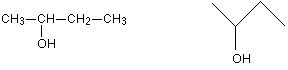In a skeletal diagram of this sort

• there is a carbon atom at each junction between bonds in a chain and at the end of each bond (unless there is something else there already - like the -OH group in the example);

• there are enough hydrogen atoms attached to each carbon to make the total number of bonds on that carbon up to 4.

Beware! Diagrams of this sort take practice to interpret correctly - and may well not be acceptable to your examiners (see below).

There are, however, some very common cases where they are frequently used. These cases involve rings of carbon atoms which are surprisingly awkward to draw tidily in a normal structural formula. Cyclohexane, C6H12, is a ring of carbon atoms each with two hydrogens attached. This is what it looks like in both a structural formula and a skeletal formula.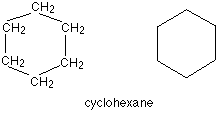And this is cyclohexene, which is similar but contains a double bond: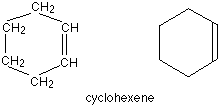But the commonest of all is the benzene ring, C6H6, which has a special symbol of its own.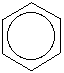## Deciding which sort of formula to use

There's no easy, all-embracing answer to this problem. It depends more than anything else on experience - a feeling that a particular way of writing a formula is best for the situation you are dealing with.

Don't worry about this - as you do more and more organic chemistry, you will probably find it will come naturally. You'll get so used to writing formulae in reaction mechanisms, or for the structures for isomers, or in simple chemical equations, that you won't even think about it.

## Contributors

Jim Clark (Chemguide.co.uk)